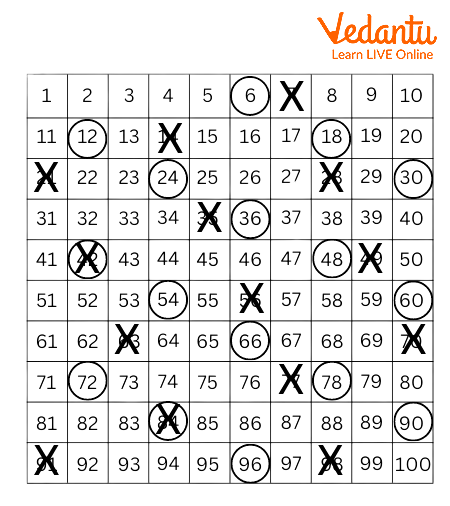Courses
Courses for Kids
Free study material
Free LIVE classes
More

# Common Multiples of 2 and 3LIVE
Join Vedantu’s FREE Mastercalss

## Introduction:

When two numbers are multiplied together, the result is a multiple, or product. For instance, 20 is a multiple of 4 and 5 if we declare that 4 multiplied by 5 will give 20. The remaining multiples of four can be written as follows: 4, 8, 12, and so on as all of them are divisible by 4. Four times one equals four.

## Common Multiples :

A whole number that is a shared multiple of every set of numbers is known as a common multiple. The term "common multiples" refers to the multiples that exist for two or more different numbers. On a grid of 100, let's mark the multiples of 6 and 7. The multiples of 6 will be marked with a circle, and the multiples of 7 with a cross.Example of Common Multiples

## Properties of Multiples :

The multiples' properties are listed below.

• All numbers are multiples of one .

• A number has an unlimited number of multiples.

• A number's multiple is greater than or equal to the original number (except for 0).

## How to Find Multiples of a Number:

When we multiply one whole number by another whole number, we have multiples as the result. Or, to put it simply, when you multiply it by a whole number, you receive its multiples.

Do you remember multiplication tables? They will be used by us to locate multiples. Let's identify the first five multiples of 6 that are not zero to see how that aids in our understanding of what multiples are.

Thus , Multiple of a number = Number Any integer (not a fraction).

## Common Multiples of 2 and 3:

The multiples of 2 are 2, 4, 6, 8, 10, 12, 14, 16, 18, 20, …

The multiples of 3 are 3, 6, 9, 12, 15, 18, 21, ….

Hence, some of the common multiples of 2 and 3 are 6, 12, 18, etc.

## Common Multiples of 3 and 5

The multiples of 3 are 3,6,9,12,15,18,21,24,27,30…..

The multiples of 5 are 5,10,15,20,25,30,35,40,45….

Hence, some common multiples of 3 and 5 are 15,30,45 etc

## Conclusion :

A common multiple is a whole number that occurs multiple times in each set of numbers. A common multiple is a multiple that two or more numbers share.

## Solved Examples:

1. List the first two multiples of 5 and 4 .

4, 8, 12, 16, 20, 24, 28, 32, 36, and 40,… are multiples of 4.

5, 10, 15, 20, 25, 30, 35, 40, and 45,... are multiples of 5.

20 and 40 are the two equivalent multiples of 5 and 4 respectively.

2. Find the least common multiples (LCM) of 3, 5 and 15.

First Let's list the multiples of 3, 5, and 15 that are common before determining the least common multiple.

Numerous multiples of 3 include 3, 6, 9, 12, 15, 18, 21, 24, 27, 30, and so forth.

5, 10, 15, 20, 25, 30, 35, 40, 45, and so on are examples of multiples of 5.

15, 30, 45, 60, 75, 90, 105, and other multiples of 15

15, 30,... and so on are common multiples of 3, 5, and 15 respectively. The smallest multiple among these regular multiples is 15. Therefore, the LCM of 3, 5, and 15 is 15 because it is the least of all the common multiples. Thus, 15 is the LCM of 3, 5 and 15.

3. List the first three multiples of 2 and 6 that are frequently repeated.

Numerous 2's include 2, 4, 6, 8, 10, 12, 14, 16, 18, 20, 22, and 24.

Numerous 6 include: 6, 12, 18, 24, 30, 36, 42, and 48.

The first two common multiples of 2 and 6 are 6 and 12.

Last updated date: 03rd Oct 2023
Total views: 68.4k
Views today: 0.68k

## FAQs on Common Multiples of 2 and 3

1. The most common multiples of 2 and 3 are.

The most common multiples of 2 are 2, 4, 6, 8, 10, 12. Common Multiples of  3 are  3, 6, 9, 12, 15, and 18.

2. How can common multiples be explained?

A whole number that is a shared multiple of every set of numbers is known as a common multiple. The term "common multiples" refers to the multiples that exist for two or more different numbers.

3. Do all numbers share a multiple in common?

A number may be multiplied by an infinite (limitless) number of factors. Therefore, there are an endless number of common multiples between any two integers or collection of numbers.

4. What connection exists between multiples and factors?

A multiple is a number that, up to a given number of times, can be divided by another number without leaving a leftover. A factor is a pair of integers or more that divide a given number by itself without leaving a residue.

5. How are multiples used in everyday life?

We frequently employ factors and multiples in our daily lives. When we want to arrange items in different ways, we employ factors.